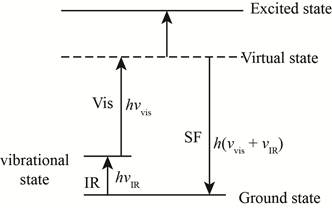# The definition of virtual state is to be stated. Concept introduction: In Raman scattering the states that generally exist are: Ground state Virtual state Excited state### Principles of Instrumental Analysis

7th Edition
Douglas A. Skoog + 2 others
Publisher: Cengage Learning
ISBN: 9781305577213### Principles of Instrumental Analysis

7th Edition
Douglas A. Skoog + 2 others
Publisher: Cengage Learning
ISBN: 9781305577213

#### Solutions

Chapter 18, Problem 18.1QAP
Interpretation Introduction

## Interpretation:The definition of virtual state is to be stated.Concept introduction:In Raman scattering the states that generally exist are:Ground state Virtual state Excited state

Expert Solution

The imaginary state is actually used to couple the ground state and excited states as a reference is known as virtual state.

### Explanation of Solution

In Raman scattering the states that generally exist are:

• Ground state
• Virtual state
• Excited state

The ground state and excited states are the real states while the virtual state is an imaginary state. This imaginary state is actually used to couple the ground state and excited states as a reference.

In the below figure virtual stage is shown.Figure-(1)

This involves the interaction of two simultaneous photons where the transition will be at the same level.

On the basis of the frequency of radiation from the source, the energy of the molecule can taken as any of an infinite number of values (virtual states) in between the ground state and the excited state.

### Want to see more full solutions like this?

Subscribe now to access step-by-step solutions to millions of textbook problems written by subject matter experts!

### Want to see more full solutions like this?

Subscribe now to access step-by-step solutions to millions of textbook problems written by subject matter experts!